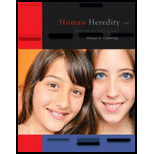# Variations on a Theme by Mendel What are the possible genotypes for the following blood types? a. type A b. type B c. type O d. type AB### Human Heredity: Principles and Iss...

11th Edition
Michael Cummings
Publisher: Cengage Learning
ISBN: 9781305251052

#### Solutions

Chapter
Section### Human Heredity: Principles and Iss...

11th Edition
Michael Cummings
Publisher: Cengage Learning
ISBN: 9781305251052
Chapter 3, Problem 32QP
Textbook Problem
1 views

## Variations on a Theme by MendelWhat are the possible genotypes for the following blood types? a. type A b. type B c. type O d. type AB

a.

Summary Introduction

To determine: The possible genotypes of the individuals with blood group A.

Introduction: The human blood group is an example of codominance where the alleles for the two antigens A and B are equally dominant. This means that when the two antigens A and B are present the blood group is AB while in the absence of both the antigens the blood group is O.

### Explanation of Solution

The genotype for the individual with blood group A can be “IA IA” or “IA i”. This is because the IA allele is dominant over “i” allele and therefore in the ho...

b.

Summary Introduction

To determine: The possible genotypes of the individual with blood group B.

Introduction: The human blood group is an example of codominance where the alleles for the two antigens A and B are equally dominant. This means that when the two antigens A and B are present the blood group is AB while in the absence of both the antigens the blood group is O.

c.

Summary Introduction

To determine: The possible genotypes of the individual with blood group O.

Introduction: The human blood group is an example of codominance where the alleles for the two antigens A and B are equally dominant. This means that when the two antigens A and B are present the blood group is AB while in the absence of both the antigens the blood group is O.

c.

Summary Introduction

To determine: The possible genotypes of the individual with blood group AB.

Introduction: The human blood group is an example of codominance where the alleles for the two antigens A and B are equally dominant. This means that when the two antigens A and B are present the blood group is AB while in the absence of both the antigens the blood group is O.

### Still sussing out bartleby?

Check out a sample textbook solution.

See a sample solution

#### The Solution to Your Study Problems

Bartleby provides explanations to thousands of textbook problems written by our experts, many with advanced degrees!

Get Started